# opencv6.5-imgproc图像处理模块之轮廓

1、图像中找轮廓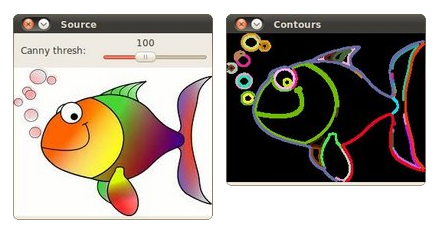/// 转成灰度并模糊化降噪
cvtColor( src, src_gray, CV_BGR2GRAY );
blur( src_gray, src_gray, Size(3,3) );
Mat canny_output;//找到轮廓的图
vector<vector<Point> > contours;//装载曲线的点
vector<Vec4i> hierarchy;

/// 用Canny算子检测边缘
Canny( src_gray, canny_output, thresh, thresh*2, 3 );
/// 寻找轮廓
findContours( canny_output, contours, hierarchy, CV_RETR_TREE, CV_CHAIN_APPROX_SIMPLE, Point(0, 0) );

/// 绘出轮廓
Mat drawing = Mat::zeros( canny_output.size(), CV_8UC3 );
for( int i = 0; i< contours.size(); i++ )//通过对contours.size(），就知道有几个分离的轮廓了
{
Scalar color = Scalar( rng.uniform(0, 255), rng.uniform(0,255), rng.uniform(0,255) );
drawContours( drawing, contours, i, color, 2, 8, hierarchy, 0, Point() );//画出轮廓
}

/// 在窗体中显示结果
namedWindow( "Contours", CV_WINDOW_AUTOSIZE );
imshow( "Contours", drawing );

void findContours(InputOutputArray image, OutputArrayOfArrays contours, int mode, int method, Point offset=Point())

contours：检测到的轮廓，每个轮廓都是存储为点向量；

hierarchy：可选的输出向量，包含图像拓扑信息。它有着和轮廓数量一样多的元素。对于第i 个轮廓contours[i]，元素hierarchy【i】【0】，hiearchy【i】【1】，hiearchy【i】【2】和hiearchy【i】【3】是在contours中基于0索引的，分别表示在同一个层次级别上的下一个轮廓上一个轮廓，第一个孩子轮廓父亲轮廓。如果轮廓 i 没有下一个、上一个、父亲或者嵌套的轮廓，对应的hierarchy【i】的元素就是负的。（这里其实就是个树结构，来进行索引不同的轮廓）

mode：轮廓索引模型

– CV_RETR_EXTERNAL     只检索最外面的轮廓。它会对所有的轮廓设置 hierarchy[i] = hierarchy[i] = -1 .
– CV_RETR_LIST                 不建立任何层次关系来进行索引所有的轮廓.
– CV_RETR_CCOMP       索引所有的轮廓并将它们组织成一个two-level的hierarchy（两个层次的层级关系）：在顶层上，有着成分的外部边界；在第二层是孔洞的边界。如果有另一个轮廓在一个连接起来的成分内部，那么就将它放在顶层上。
– CV_RETR_TREE        检索所有的轮廓并重构一个全层次的嵌套轮廓结构，在contours.c的例子中你更可以看到全层次建立的代码。
method ：轮廓逼近的方法

– CV_CHAIN_APPROX_NONE   存储所有轮廓点的绝对值。也就是说任何的轮廓的2子序列点 (x1,y1) 和 (x2,y2) 就是表示水平的，竖直的或者对角线邻居，也就是： max(abs(x1-x2),abs(y2-y1))==1；
– CV_CHAIN_APPROX_SIMPLE    压缩水平的，竖直的和对角线线段，只保留他们的终端。例如：对于一个up-right 的矩形轮廓是由4个点进行编码的。

– CV_CHAIN_APPROX_TC89_L1，  CV_CHAIN_APPROX_TC89_KCOS     使用 Teh-Chin 链逼近算法中主流之一的算法。

offset：可选的偏移量，通过这个值可以平移每一个轮廓。当轮廓是从一个图像的ROI中提取而你需要在整个图像上下文中分析的时候会变得很有用。

Notes:image会被该函数所修改，同样的该函数不考虑图像的1像素边界（它会被0填充然后用来作为邻居分析），因此接触图像边界的轮廓会被修剪（clip，夹）。

第一个参数：目标图像，即画布；

第二个参数：所有的输入轮廓，每个轮廓存储成点向量的形式；

第三个参数：用来索引需要画的轮廓的参数，如果是负的，那么就画所有的轮廓；

第四个参数：轮廓的颜色；

第五个参数：画的轮廓的线的粗细程度，如果是负的（例如：thickness = CV_FILLED），轮廓内部也会画出来；

第六个参数：线连接类型，line（）可以有更详细的说明。

第七个参数：可选的关于层次的信息。当你只想画某些轮廓的时候才是需要的（见maxLevel）；

第八个参数：画轮廓的最大等级。如果为0，那么只画指定的轮廓。如果为1，该函数会画轮廓及所有的嵌套轮廓。如果为2，该函数会画轮廓、所有的嵌套轮廓、所有的嵌套-to-嵌套的轮廓，以此类推。该参数当hierarchy可用的时候才被考虑；

第九个参数：可选的轮廓平移参数。通过制定的平移量offset = （dx，dy）来平移所有的画的轮廓。

Mat src;
src = imread（name，0）;
Mat dst = Mat::zeros(src.rows, src.cols, CV_8UC3);
src = src > 1;

vector<vector<Point> > contours;
vector<Vec4i> hierarchy;
findContours( src, contours, hierarchy,RETR_CCOMP, CHAIN_APPROX_SIMPLE );
//通过对top-level的轮廓进行迭代来画出每个联通的成分，使用随机颜色

int idx = 0;
for( ; idx >= 0; idx = hierarchy[idx] ){
Scalar color( rand()&255, rand()&255, rand()&255 );
drawContours( dst, contours, idx, color, FILLED, 8, hierarchy );
}
namedWindow( "Components", 1 );
imshow( "Components", dst );
waitKey(0);
2、计算物体的凸包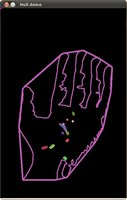/// 转成灰度图并进行模糊降噪
cvtColor( src, src_gray, CV_BGR2GRAY );
blur( src_gray, src_gray, Size(3,3) );
Mat src_copy = src.clone();
Mat threshold_output;
vector<vector<Point> > contours;//存储轮廓的点集合
vector<Vec4i> hierarchy;//构建轮廓的层次结构

/// 对图像进行二值化
int thresh = 100;
int max_thresh = 255;
RNG rng(12345);   threshold( src_gray, threshold_output, thresh, 255, THRESH_BINARY );   /// 寻找轮廓   findContours( threshold_output, contours, hierarchy, CV_RETR_TREE, CV_CHAIN_APPROX_SIMPLE, Point(0, 0) );   /// 对每个轮廓计算其凸包   vector<vector<Point> >hull( contours.size() );   for( int i = 0; i < contours.size(); i++ )      {  
     convexHull( Mat(contours[i]), hull[i], false );//凸包计算
     }

/// 绘出轮廓及其凸包
Mat drawing = Mat::zeros( threshold_output.size(), CV_8UC3 );
for( int i = 0; i< contours.size(); i++ )
{
Scalar color = Scalar( rng.uniform(0, 255), rng.uniform(0,255), rng.uniform(0,255) );
drawContours( drawing, contours, i, color, 1, 8, vector<Vec4i>(), 0, Point() );//画轮廓
drawContours( drawing, hull, i, color, 1, 8, vector<Vec4i>(), 0, Point() );//画凸包
}

/// 把结果显示在窗体
namedWindow( "Hull demo", CV_WINDOW_AUTOSIZE );
imshow( "Hull demo", drawing );

该函数使用Sklansky的算法来查找一个2d点集的凸包，在当前的执行情况下的复杂度是O(NlogN)，可以参见convexhull.cpp中验证不同的函数变量的结果。

3、给轮廓加上矩形或者圆形边界框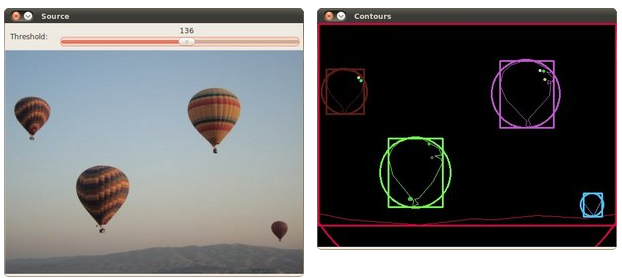int thresh = 100;
int max_thresh = 255;
RNG rng(12345);
/// 转化成灰度图像并进行平滑 用来减少噪音点
cvtColor( src, src_gray, CV_BGR2GRAY );
blur( src_gray, src_gray, Size(3,3) );
Mat threshold_output;
vector<vector<Point> > contours;//存储轮廓点
vector<Vec4i> hierarchy;//构建不同轮廓的层次结构

/// 使用Threshold检测边缘
threshold( src_gray, threshold_output, thresh, 255, THRESH_BINARY );
/// 找到轮廓
findContours( threshold_output, contours, hierarchy, CV_RETR_TREE, CV_CHAIN_APPROX_SIMPLE, Point(0, 0) );

/// 多边形逼近轮廓 + 获取矩形和圆形边界框
vector<vector<Point> > contours_poly( contours.size() );//创建contours.size()个空的多边形
vector<Rect> boundRect( contours.size() );//创建contours.size()个矩形框
vector<Point2f>center( contours.size() );//创建contours.size()个圆心

for( int i = 0; i < contours.size(); i++ )
{ 
       approxPolyDP( Mat(contours[i]), contours_poly[i], 3, true );//多边形逼近
boundRect[i] = boundingRect( Mat(contours_poly[i]) );//获取某个轮廓的矩形框
}

/// 画多边形轮廓 + 包围的矩形框 + 圆形框
Mat drawing = Mat::zeros( threshold_output.size(), CV_8UC3 );
for( int i = 0; i< contours.size(); i++ )
{
Scalar color = Scalar( rng.uniform(0, 255), rng.uniform(0,255), rng.uniform(0,255) );//随机颜色
drawContours( drawing, contours_poly, i, color, 1, 8, vector<Vec4i>(), 0, Point() );//画轮廓-多边形
rectangle( drawing, boundRect[i].tl(), boundRect[i].br(), color, 2, 8, 0 );//画矩形
circle( drawing, center[i], (int)radius[i], color, 2, 8, 0 );//画圆形
}

/// 显示在一个窗口
namedWindow( "Contours", CV_WINDOW_AUTOSIZE );
imshow( "Contours", drawing );

– std::vector or Mat    (C++ interface)
– Nx2 numpy array      (Python interface)
– CvSeq or ‘‘ CvMat      (C interface)

– std::vector<> or Mat (C++ interface)
– CvSeq* or CvMat* (C interface)
– Nx2 numpy array (Python interface)

4、给轮廓加上倾斜的边界框和椭圆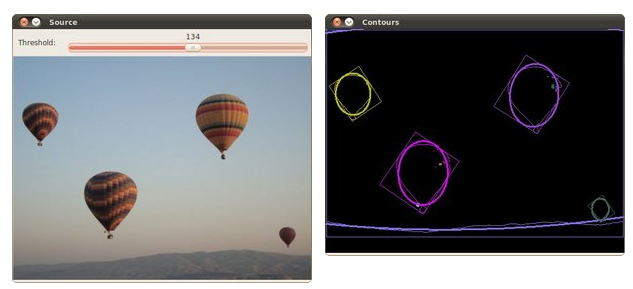int thresh = 100;
int max_thresh = 255;
RNG rng(12345);
 /// 转为灰度图并模糊化 来减小噪音点
cvtColor( src, src_gray, CV_BGR2GRAY );
blur( src_gray, src_gray, Size(3,3) );

 Mat threshold_output;
vector<vector<Point> > contours;//存储轮廓的向量
vector<Vec4i> hierarchy;//轮廓的层次结构

/// 阈值化检测边界
threshold( src_gray, threshold_output, thresh, 255, THRESH_BINARY );
/// 寻找轮廓
findContours( threshold_output, contours, hierarchy, CV_RETR_TREE, CV_CHAIN_APPROX_SIMPLE, Point(0, 0) );

/// 对每个找到的轮廓创建可倾斜的边界框和椭圆
vector<RotatedRect> minRect( contours.size() );//存储contours.size()个旋转的边界框***************此处是重点，RotateRect
vector<RotatedRect> minEllipse( contours.size() );////存储contours.size()个旋转的椭圆*************

for( int i = 0; i < contours.size(); i++ )
{ 
        minRect[i] = minAreaRect( Mat(contours[i]) );//获取包含最小区域的矩形
if( contours[i].size() > 5 )
{ 
         minEllipse[i] = fitEllipse( Mat(contours[i]) );//获取椭圆所需的信息
         }
}

/// 绘出轮廓及其可倾斜的边界框和边界椭圆
Mat drawing = Mat::zeros( threshold_output.size(), CV_8UC3 );
for( int i = 0; i< contours.size(); i++ )
{
Scalar color = Scalar( rng.uniform(0, 255), rng.uniform(0,255), rng.uniform(0,255) );
// contour
drawContours( drawing, contours, i, color, 1, 8, vector<Vec4i>(), 0, Point() );//画对象的轮廓
// ellipse
ellipse( drawing, minEllipse[i], color, 2, 8 );//画椭圆
// rotated rectangle
Point2f rect_points; 
       minRect[i].points( rect_points );//将旋转矩形的顶点赋值给实参；
for( int j = 0; j < 4; j++ )
line( drawing, rect_points[j], rect_points[(j+1)%4], color, 1, 8 );//画出倾斜矩形，采用的是画四条线的形式实现的
}

/// 结果在窗体中显示
namedWindow( "Contours", CV_WINDOW_AUTOSIZE );
imshow( "Contours", drawing );

– std::vector<> or Mat (C++ interface)

– CvSeq* or CvMat* (C interface)
– Nx2 numpy array (Python interface)

– std::vector<> or Mat (C++ interface)
– CvSeq* or CvMat* (C interface)
– Nx2 numpy array (Python interface)

5、计算轮廓的矩

int thresh = 100;
int max_thresh = 255;
RNG rng(12345);
 /// 把原图像转化成灰度图像并进行平滑
cvtColor( src, src_gray, CV_BGR2GRAY );
blur( src_gray, src_gray, Size(3,3) );
Mat canny_output;
vector<vector<Point> > contours;//存储轮廓
vector<Vec4i> hierarchy;//存储轮廓的层次结构

/// 使用Canndy检测边缘
Canny( src_gray, canny_output, thresh, thresh*2, 3 );
/// 找到轮廓
findContours( canny_output, contours, hierarchy, CV_RETR_TREE, CV_CHAIN_APPROX_SIMPLE, Point(0, 0) );

/// 计算矩
vector<Moments> mu(contours.size() );
for( int i = 0; i < contours.size(); i++ )
{ 
         mu[i] = moments( contours[i], false );//计算轮廓的矩
      }

///  计算中心矩:
vector<Point2f> mc( contours.size() );
for( int i = 0; i < contours.size(); i++ )
{ 
          mc[i] = Point2f( mu[i].m10/mu[i].m00 , mu[i].m01/mu[i].m00 );
      }

/// 绘制轮廓
Mat drawing = Mat::zeros( canny_output.size(), CV_8UC3 );
for( int i = 0; i< contours.size(); i++ )
{
Scalar color = Scalar( rng.uniform(0, 255), rng.uniform(0,255), rng.uniform(0,255) );
drawContours( drawing, contours, i, color, 2, 8, hierarchy, 0, Point() );
circle( drawing, mc[i], 4, color, -1, 8, 0 );
}

/// 显示到窗口中
namedWindow( "Contours", CV_WINDOW_AUTOSIZE );
imshow( "Contours", drawing );

/// 通过m00计算轮廓面积并且和OpenCV函数比较
printf("\t Info: Area and Contour Length \n");
for( int i = 0; i< contours.size(); i++ )
{
printf(" * Contour[%d] - Area (M_00) = %.2f - Area OpenCV: %.2f - Length: %.2f \n", 
              i, mu[i].m00, contourArea(contours[i]), arcLength( contours[i], true ) //第几个轮廓，轮廓的空间矩，轮廓的面积，轮廓的周长
               );//计算轮廓面积和 轮廓或曲线的长度
Scalar color = Scalar( rng.uniform(0, 255), rng.uniform(0,255), rng.uniform(0,255) );
drawContours( drawing, contours, i, color, 2, 8, hierarchy, 0, Point() );
circle( drawing, mc[i], 4, color, -1, 8, 0 );


class Moments
{
public:
<span style="white-space:pre">		</span>Moments();
<span style="white-space:pre">		</span>Moments(double m00, double m10, double m01, double m20, double m11,
<span style="white-space:pre">		</span>double m02, double m30, double m21, double m12, double m03 );
<span style="white-space:pre">		</span>Moments( const CvMoments& moments );
<span style="white-space:pre">		</span>operator CvMoments() const;
// spatial moments
<span style="white-space:pre">		</span>double m00, m10, m01, m20, m11, m02, m30, m21, m12, m03;
// central moments
<span style="white-space:pre">		</span>double mu20, mu11, mu02, mu30, mu21, mu12, mu03;
// central normalized moments
<span style="white-space:pre">		</span>double nu20, nu11, nu02, nu30, nu21, nu12, nu03;
}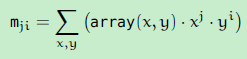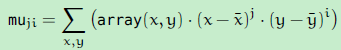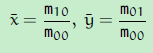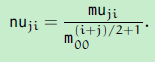notes：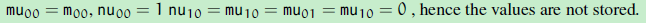vector<Point> contour;
contour.push_back(Point2f(0, 0));
contour.push_back(Point2f(10, 0));
contour.push_back(Point2f(10, 10));
contour.push_back(Point2f(5, 4));
double area0 = contourArea(contour);
vector<Point> approx;
approxPolyDP(contour, approx, 5, true);
double area1 = contourArea(approx);
cout << "area0 =" << area0 << endl <<
<span style="white-space:pre">	</span>"area1 =" << area1 << endl <<
<span style="white-space:pre">	</span>"approx poly vertices" << approx.size() << endl;

6、多边形测试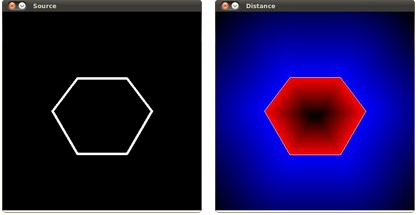/// 创建一个图形     const int r = 100;
Mat src = Mat::zeros( Size( 4*r, 4*r ), CV_8UC1 );//创建画布

/// 绘制一系列点创建一个轮廓:
vector<Point2f> vert(6);//一个有6个点的轮廓

vert = Point( 1.5*r, 1.34*r );
vert = Point( 1*r, 2*r );
vert = Point( 1.5*r, 2.866*r );
vert = Point( 2.5*r, 2.866*r );
vert = Point( 3*r, 2*r );
vert = Point( 2.5*r, 1.34*r );//自己定轮廓的6各点

/// 在src内部绘制
for( int j = 0; j < 6; j++ ){ 
      line( src, vert[j],  vert[(j+1)%6], Scalar( 255 ), 3, 8 ); //将该轮廓画出来
      }

/// 得到轮廓
vector<vector<Point> > contours; vector<Vec4i> hierarchy;
Mat src_copy = src.clone();

findContours( src_copy, contours, hierarchy, RETR_TREE, CHAIN_APPROX_SIMPLE);//查找轮廓

/// 计算到轮廓的距离
Mat raw_dist( src.size(), CV_32FC1 );

for( int j = 0; j < src.rows; j++ ){ 
    for( int i = 0; i < src.cols; i++ ){
           raw_dist.at<float>(j,i) = pointPolygonTest( contours, Point2f(i,j), true ); 整个画布，逐点测试到第0个轮廓的距离
          }
}

double minVal; double maxVal;
minMaxLoc( raw_dist, &minVal, &maxVal, 0, 0, Mat() );//查找全局最大最小
minVal = abs(minVal); maxVal = abs(maxVal);

/// 图形化的显示距离
Mat drawing = Mat::zeros( src.size(), CV_8UC3 );

for( int j = 0; j < src.rows; j++ ){ 
      for( int i = 0; i < src.cols; i++ ) {
if( raw_dist.at<float>(j,i) < 0 ){ 
               drawing.at<Vec3b>(j,i) = 255 - (int) abs(raw_dist.at<float>(j,i))*255/minVal;
             }
else if( raw_dist.at<float>(j,i) > 0 ){
               drawing.at<Vec3b>(j,i) = 255 - (int) raw_dist.at<float>(j,i)*255/maxVal;
              }
else{ 
              drawing.at<Vec3b>(j,i) = 255; drawing.at<Vec3b>(j,i) = 255; drawing.at<Vec3b>(j,i) = 255;
              }
}
}

/// 创建窗口显示结果
char* source_window = "Source";
namedWindow( source_window, CV_WINDOW_AUTOSIZE );
imshow( source_window, src );
namedWindow( "Distance", CV_WINDOW_AUTOSIZE );
imshow( "Distance", drawing );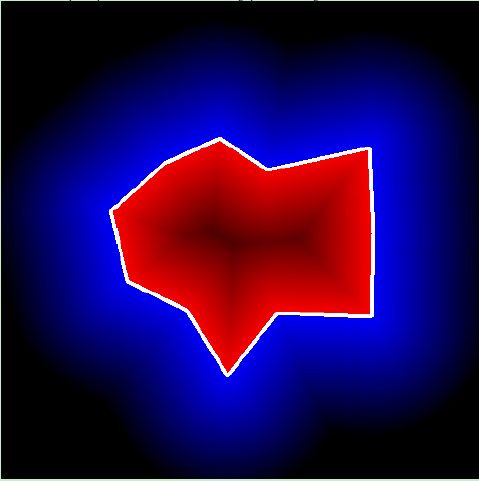posted @ 2015-01-13 22:18  仙守  阅读(2910)  评论(0编辑  收藏  举报